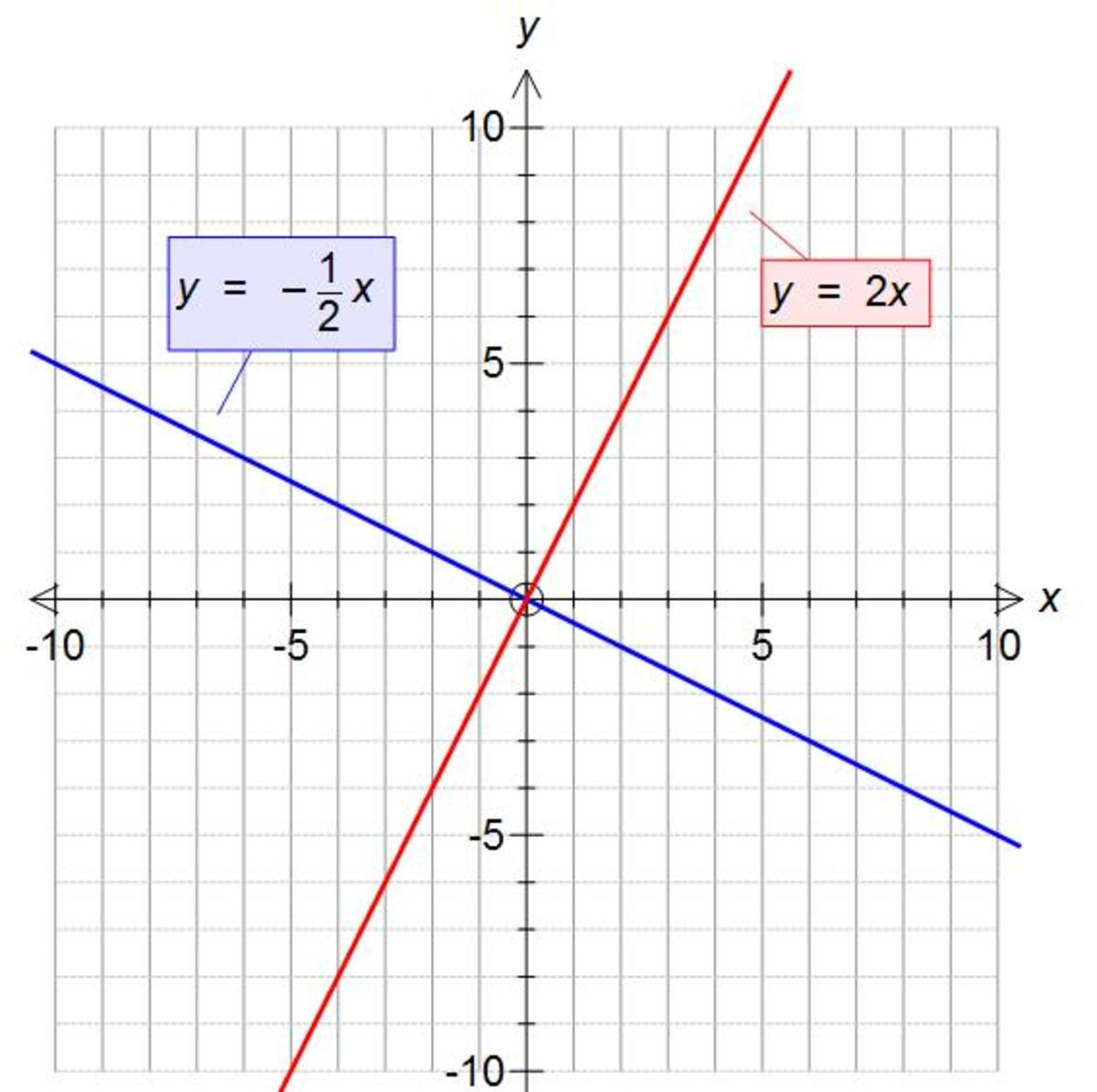Updated date:

# Perpendicular gradients. Calculating the perpendicular gradient of a linear graph.

Author:A perpendicular line is a line that is at right angles to another line. If you multiply the gradients of these lines together you will get -1. So if the gradient of the first line is m then the gradient of the second line is -1/m:

Perpendicular gradient = -1/m

Take a look at the two lines in the picture which are perpendicular to each other. The gradient of the red line is 2 and the gradient of the blue line is -0.5. If you multiply these two numbers together you get -1:

2 × -0.5 = -1

Let’s take a look at a few example on working out the perpendicular gradient of lines.

Example 1

The equation of a line is y = 3x. Work out the gradient of the line perpendicular to this line.

The gradient of the line given is 3, so m = 3 (as the coefficient of x is 3)

All you need to do now is use the formula given above the working out the perpendicular gradient:

Perpendicular gradient = -1/m

Perpendicular gradient = -1/3

So the gradient of the perpendicular line is -1/3

Example 2

The equation of a line is y = 5x - 1. Work out the gradient of the line perpendicular to this line.

The gradient of the line given is 5, so m = 5 (as the coefficient of x is 5)

All you need to do now is use the formula given above the working out the perpendicular gradient:

Perpendicular gradient = -1/m

Perpendicular gradient = -1/5

So the gradient of the perpendicular line is -1/5

Example 3

The equation of a line is y = -2x + 4. Work out the gradient of the line perpendicular to this line.

The gradient of the line given is -2, so m = -2 (as the coefficient of x is -2)

All you need to do now is use the formula given above the working out the perpendicular gradient:

Perpendicular gradient = -1/m

Perpendicular gradient = -1/-2 = 1/2

So the gradient of the perpendicular line is 1/2

Example 4

The equation of a line is y = ¼x - 6. Work out the gradient of the line perpendicular to this line.

The gradient of the line given is ¼ so m = ¼ (as the coefficient of x is ¼ )

All you need to do now is use the formula given above the working out the perpendicular gradient:

Perpendicular gradient = -1/m

Perpendicular gradient = -1/0.25 = -4

So the gradient of the perpendicular line is -4